# Scientific Python Quickstart¶

#### ANU¶

This is a fast-paced, hands-on introduction to scientific computing with Python, contained in a Jupyter notebook. The main focus will be on introducing Python's four most important scientific libraries: NumPy, Scipy, Pandas and Matplotlib.

If you don't know how to use this notebook you need to first work through this page.

A slower, more detailed and more systematic treatment of Python for scientific applications can be found at quant-econ.net. But this notebook is a good place to start for those who like to learn by doing.

Here's some information on the version of Python that I'm using:

In :
import sys
print(sys.version)

3.5.1 |Anaconda 2.4.1 (64-bit)| (default, Dec  7 2015, 11:16:01)
[GCC 4.4.7 20120313 (Red Hat 4.4.7-1)]


## Basic NumPy¶

Perhaps the single most important scientific library for Python is NumPy. NumPy provides foundational data structures and routines on which many other libraries rely.

In :
import numpy as np     # Import library and give it alias np
print(np.__version__)  #  The version I'm using

1.10.2


NumPy defines a basic data type called an array (actually a numpy.ndarray)

In :
a = np.zeros(3)     # Create an array of zeros
a                   # Print a

Out:
array([ 0.,  0.,  0.])
In :
type(a)

Out:
numpy.ndarray

Note that array data must be homogeneous

The most important data types are:

• float64: 64 bit floating point number
• float32: 32 bit floating point number
• int64: 64 bit integer
• int32: 32 bit integer
• bool: 8 bit True or False

There are also dtypes to represent complex numbers, unsigned integers, etc

On most machines, the default dtype for arrays is float64

In :
a = np.zeros(3)
type(a)

Out:
numpy.float64

When we create an array such as

In :
z = np.zeros(10)


z is a "flat" array with no dimension--- neither row nor column vector:

In :
 z.shape

Out:
(10,)

Here the shape tuple has only one element, which is the length of the array (tuples with one element end with a comma)

To give it dimension, we can change the shape attribute

For example, let's make it a column vector

In :
z.shape = (10, 1)

In :
z

Out:
array([[ 0.],
[ 0.],
[ 0.],
[ 0.],
[ 0.],
[ 0.],
[ 0.],
[ 0.],
[ 0.],
[ 0.]])
In :
z = np.zeros(4)
z.shape = (2, 2)
z

Out:
array([[ 0.,  0.],
[ 0.,  0.]])

### Creating arrays¶

Creating empty arrays --- initializing memory:

In :
z = np.empty(3)
z

Out:
array([ 0.,  0.,  0.])

These are just garbage numbers --- whatever was in those memory slots

Here's how to make a regular gird sequence

In :
z = np.linspace(2, 4, 5)  # From 2 to 4, with 5 elements
z

Out:
array([ 2. ,  2.5,  3. ,  3.5,  4. ])

Creating an array of ones

In :
z = np.ones(3)
z

Out:
array([ 1.,  1.,  1.])
In :
z = np.identity(2)
z

Out:
array([[ 1.,  0.],
[ 0.,  1.]])

Arrays can be made from Python lists or tuples

In :
z = np.array([10, 20])
z

Out:
array([10, 20])
In :
z = np.array((10, 20), dtype=float)
z

Out:
array([ 10.,  20.])
In :
z = np.array([[1, 2], [3, 4]])         # 2D array from a list of lists
z

Out:
array([[1, 2],
[3, 4]])

### Array indexing¶

In :
z = np.linspace(1, 2, 5)
z

Out:
array([ 1.  ,  1.25,  1.5 ,  1.75,  2.  ])
In :
z  # First element --- Python sequences are zero based, like C, Java, etc.

Out:
1.0
In :
z[-1]  # Special syntax for last element

Out:
2.0
In :
z[0:2]  # Meaning: Two elements, starting from element 0

Out:
array([ 1.  ,  1.25])
In :
z = np.array([[1, 2], [3, 4]])
z

Out:
array([[1, 2],
[3, 4]])
In :
z[0, 0]

Out:
1
In :
z[0,:]  # First row

Out:
array([1, 2])
In :
z[:,0]  # First column

Out:
array([1, 3])
In :
z = np.linspace(2, 4, 5)
z

Out:
array([ 2. ,  2.5,  3. ,  3.5,  4. ])
In :
d = np.array([0, 1, 1, 0, 0], dtype=bool)
d

Out:
array([False,  True,  True, False, False], dtype=bool)
In :
z[d]

Out:
array([ 2.5,  3. ])

### Array methods¶

In :
A = np.array((4, 3, 2, 1))
A

Out:
array([4, 3, 2, 1])
In :
A.sort()

In :
A

Out:
array([1, 2, 3, 4])
In :
A.mean()

Out:
2.5
In :
A.sum()

Out:
10
In :
A.max()

Out:
4
In :
A.cumsum()

Out:
array([ 1,  3,  6, 10])
In :
A.var()

Out:
1.25
In :
A.shape = (2, 2)
A

Out:
array([[1, 2],
[3, 4]])
In :
A.T  # Transpose, equivalent to A.transpose()

Out:
array([[1, 3],
[2, 4]])

### Operations on arrays¶

Standard arithmetic operations on arrays act elementwise

In :
a = np.array([1, 2, 3, 4])
b = np.array([5, 6, 7, 8])

In :
a + b

Out:
array([ 6,  8, 10, 12])
In :
a - b

Out:
array([-4, -4, -4, -4])
In :
a + 10

Out:
array([11, 12, 13, 14])
In :
a.shape = 2, 2
b.shape = 2, 2

In :
a

Out:
array([[1, 2],
[3, 4]])
In :
b

Out:
array([[5, 6],
[7, 8]])
In :
a * b # Pointwise multiplication!!

Out:
array([[ 5, 12],
[21, 32]])
In :
np.dot(a, b) # Matrix multiplication

Out:
array([[19, 22],
[43, 50]])

For Python $\geq 3.5$ and NumPy $\geq 1.1$ the @ operator also works.

In :
a @ b

Out:
array([[19, 22],
[43, 50]])

I'll continue to use np.dot below for the benefit of those who are using older versions. But in my opinion the @ operator is much nicer.

### Comparisons¶

In :
z = np.array([2, 3])
y = np.array([2, 3])
z == y

Out:
array([ True,  True], dtype=bool)
In :
y = 3
z == y

Out:
array([False,  True], dtype=bool)
In :
z = np.linspace(0, 10, 5)
z

Out:
array([  0. ,   2.5,   5. ,   7.5,  10. ])
In :
z > 3

Out:
array([False, False,  True,  True,  True], dtype=bool)
In :
z[z > 3]  # Conditional extraction

Out:
array([  5. ,   7.5,  10. ])

## Matplotlib¶

Matplotlib is an outstanding plotting and visualization library for Python that interacts nicely with NumPy. Here are a few quick examples. We'll see more below when we discuss the SciPy library.

In :
import matplotlib.pyplot as plt  # Import main functionality


Display figures in this browser window rather than having them open up separately:

In :
%matplotlib inline


Create something to plot

In :
x = np.linspace(-2, 2, 100)
y = x**2

In :
fig, ax = plt.subplots()  # Create axes and figure window
ax.plot(x, y, 'b-')

Out:
[<matplotlib.lines.Line2D at 0x7f77a347d048>]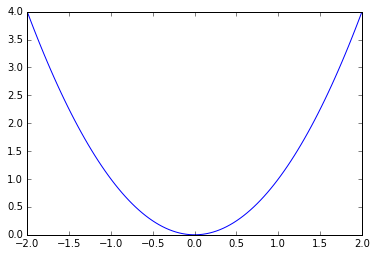Here's a slightly more complex plot

In :
y3 = x**3
fig, ax = plt.subplots()  # Create axes and figure window
ax.plot(x, y, 'b-', lw=2, alpha=0.8, label='$x^2$')
ax.plot(x, y3, 'g-', lw=2, alpha=0.8, label='$x^3$')
ax.legend(loc='lower right')

Out:
<matplotlib.legend.Legend at 0x7f77a228fcf8>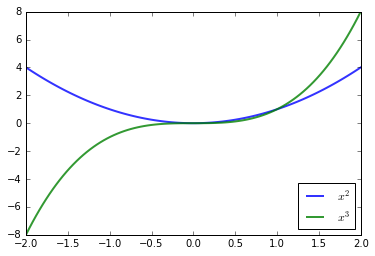## SciPy¶

Let's just cover some simple examples --- references for further reading are below

### Statistics and distributions¶

Let's use scipy.stats to generate some data from the Beta distribution

In :
from scipy.stats import beta
q = beta(5, 5)      # Beta(a, b), with a = b = 5
obs = q.rvs(2000)   # 2000 observations


Now let's histogram it and compare it to the original density

In :
fig, ax = plt.subplots()
ax.hist(obs, bins=40, normed=True)
grid = np.linspace(0.01, 0.99, 100)
ax.plot(grid, q.pdf(grid), 'k-', linewidth=2)

Out:
[<matplotlib.lines.Line2D at 0x7f77a22d2ef0>]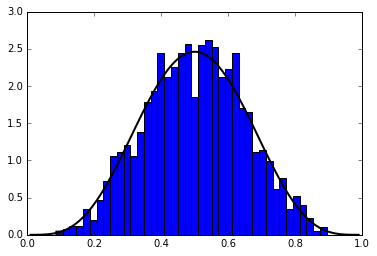Other methods

In :
type(q)

Out:
scipy.stats._distn_infrastructure.rv_frozen
In :
dir(q)  # Let's see all its methods

Out:
['__class__',
'__delattr__',
'__dict__',
'__dir__',
'__doc__',
'__eq__',
'__format__',
'__ge__',
'__getattribute__',
'__gt__',
'__hash__',
'__init__',
'__le__',
'__lt__',
'__module__',
'__ne__',
'__new__',
'__reduce__',
'__reduce_ex__',
'__repr__',
'__setattr__',
'__sizeof__',
'__str__',
'__subclasshook__',
'__weakref__',
'a',
'args',
'b',
'cdf',
'dist',
'entropy',
'expect',
'interval',
'isf',
'kwds',
'logcdf',
'logpdf',
'logpmf',
'logsf',
'mean',
'median',
'moment',
'pdf',
'pmf',
'ppf',
'random_state',
'rvs',
'sf',
'stats',
'std',
'var']
In :
q.cdf(0.5)

Out:
0.50000000000000011
In :
q.pdf(0.5)

Out:
2.4609375000000009
In :
q.mean()

Out:
0.5

Basic linear regression:

In :
from scipy.stats import linregress
n = 100
alpha, beta, sigma = 1, 2, 1.5
x = np.random.randn(n)  # n standard normals
y = alpha + beta * x + sigma * np.random.randn(n)
beta_hat, alpha_hat, r_value, p_value, std_err = linregress(x, y)
print("intercept = {}".format(alpha_hat))

gradient = 1.874172371477785
intercept = 1.4035329152756753


Let's plot this with data and line of best fit

In :
fig, ax = plt.subplots(figsize=(8, 5))
ax.plot(x, y, 'bo', alpha=0.6, label='observations')
xgrid = np.linspace(-3, 3, 2)
ax.plot(xgrid, alpha_hat + beta_hat * xgrid, 'k-', lw=2, alpha=0.8, label='best fit')
ax.grid()
ax.legend(loc='upper left')

Out:
<matplotlib.legend.Legend at 0x7f77a21026a0>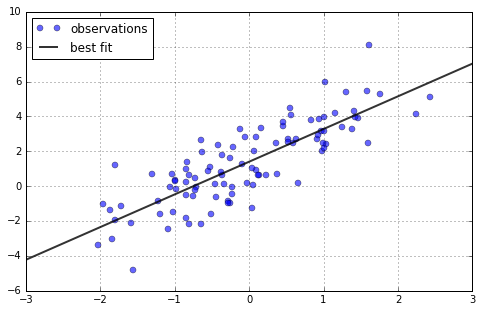### Roots and fixed points¶

Let's choose an arbitrary function to work with

In :
fig, ax = plt.subplots()
def f(x):
return np.sin(4 * (x - 0.25)) + x + x**20 - 1
x = np.linspace(0, 1, 100)
ax.plot(x, f(x))
ax.plot(x, 0 * x)

Out:
[<matplotlib.lines.Line2D at 0x7f77a2129c18>]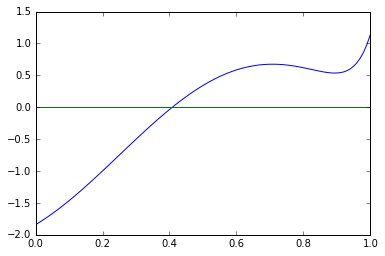In :
from scipy.optimize import bisect  # Bisection algorithm --- slow but robust
bisect(f, 0, 1)

Out:
0.4082935042797544
In :
from scipy.optimize import newton  #  Newton's method --- fast but less robust
newton(f, 0.2)   # Start the search at initial condition x = 0.2

Out:
0.40829350427935679
In :
newton(f, 0.7)   # Start the search at x = 0.7 instead

Out:
0.70017000000002816

Here we see that the algorithm gets it wrong --- newton is fast but not robust

Let's try a hybrid method

In :
from scipy.optimize import brentq
brentq(f, 0, 1) # Hybrid method

Out:
0.40829350427936706
In :
timeit bisect(f, 0, 1)

10000 loops, best of 3: 64.7 µs per loop

In :
timeit newton(f, 0.2)

100000 loops, best of 3: 14.2 µs per loop

In :
timeit brentq(f, 0, 1)

100000 loops, best of 3: 16.1 µs per loop


Note that the hybrid method is robust but still quite fast...

### Numerical optimization and integration¶

In :
from scipy.optimize import fminbound
fminbound(lambda x: x**2, -1, 2)  # Search in [-1, 2]

Out:
0.0
In :
from scipy.integrate import quad
integral, error = quad(lambda x: x**2, 0, 1)
integral

Out:
0.33333333333333337

### Linear Algebra¶

Let's look at some of the most common routines from linear and matrix algebra

In :
import scipy.linalg as la


We'll experiment with matrices

$$A = \begin{bmatrix} 2 & -1 \\ 3 & 0 \end{bmatrix} \quad \text{and} \quad b = \begin{bmatrix} 1 \\ 1 \end{bmatrix}$$
In :
A = [[2, -1],
[3, 0]]
A = np.array(A) # Convert from list to NumPy array
b = np.ones((2, 1))  # Shape is 2 x 1

In :
A

Out:
array([[ 2, -1],
[ 3,  0]])
In :
b

Out:
array([[ 1.],
[ 1.]])
In :
x = la.solve(A, b)  # Solve for x in Ax = b
print(x)

[[ 0.33333333]
[-0.33333333]]


Let's check that $Ax = b$

In :
np.dot(A, x)

Out:
array([[ 1.],
[ 1.]])

We can also invert directly

In :
la.inv(A)

Out:
array([[ 0.        ,  0.33333333],
[-1.        ,  0.66666667]])
In :
np.dot(A, la.inv(A))  # Should be the identity

Out:
array([[ 1.,  0.],
[ 0.,  1.]])

Let's compute the eigenvalues and eigenvectors

In :
eigvals, eigvecs = la.eig(A)

In :
print("eigenvalues = {}".format(eigvals))

eigenvalues = [ 1.+1.41421356j  1.-1.41421356j]

In :
print("first eigenvector = {}".format(eigvecs[:, 0]))

first eigenvector = [ 0.28867513+0.40824829j  0.86602540+0.j        ]


## Pandas¶

Pandas is a very popular library for working with data sets. In pandas, data is held in a dataframe, which is kind of like a spread sheet

In :
import pandas as pd


Let's start by writing a test data set to the present working directory, so we can read it back in as a dataframe using pandas. We use an IPython magic to write the data from a cell to a file:

In :
%%file test_data.csv
"country","country isocode","year","POP","XRAT","tcgdp","cc","cg"
"Argentina","ARG","2000","37335.653","0.9995","295072.21869","75.716805379","5.5788042896"
"Australia","AUS","2000","19053.186","1.72483","541804.6521","67.759025993","6.7200975332"
"India","IND","2000","1006300.297","44.9416","1728144.3748","64.575551328","14.072205773"
"Israel","ISR","2000","6114.57","4.07733","129253.89423","64.436450847","10.266688415"
"Malawi","MWI","2000","11801.505","59.543808333","5026.2217836","74.707624181","11.658954494"
"South Africa","ZAF","2000","45064.098","6.93983","227242.36949","72.718710427","5.7265463933"
"United States","USA","2000","282171.957","1","9898700","72.347054303","6.0324539789"
"Uruguay","URY","2000","3219.793","12.099591667","25255.961693","78.978740282","5.108067988"

Overwriting test_data.csv

In :
%ls ./*.csv # Check it's there

./test_data.csv

In :
df = pd.read_csv('./test_data.csv')

In :
df

Out:
country country isocode year POP XRAT tcgdp cc cg
0 Argentina ARG 2000 37335.653 0.999500 295072.218690 75.716805 5.578804
1 Australia AUS 2000 19053.186 1.724830 541804.652100 67.759026 6.720098
2 India IND 2000 1006300.297 44.941600 1728144.374800 64.575551 14.072206
3 Israel ISR 2000 6114.570 4.077330 129253.894230 64.436451 10.266688
4 Malawi MWI 2000 11801.505 59.543808 5026.221784 74.707624 11.658954
5 South Africa ZAF 2000 45064.098 6.939830 227242.369490 72.718710 5.726546
6 United States USA 2000 282171.957 1.000000 9898700.000000 72.347054 6.032454
7 Uruguay URY 2000 3219.793 12.099592 25255.961693 78.978740 5.108068

Let's try that again but this time using the country as the index column

In :
df = pd.read_csv('./test_data.csv', index_col='country')

In :
df

Out:
country isocode year POP XRAT tcgdp cc cg
country
Argentina ARG 2000 37335.653 0.999500 295072.218690 75.716805 5.578804
Australia AUS 2000 19053.186 1.724830 541804.652100 67.759026 6.720098
India IND 2000 1006300.297 44.941600 1728144.374800 64.575551 14.072206
Israel ISR 2000 6114.570 4.077330 129253.894230 64.436451 10.266688
Malawi MWI 2000 11801.505 59.543808 5026.221784 74.707624 11.658954
South Africa ZAF 2000 45064.098 6.939830 227242.369490 72.718710 5.726546
United States USA 2000 282171.957 1.000000 9898700.000000 72.347054 6.032454
Uruguay URY 2000 3219.793 12.099592 25255.961693 78.978740 5.108068

Let's drop the year since it's not very informative

In :
df.drop(['year'], axis=1, inplace=True)
df

Out:
country isocode POP XRAT tcgdp cc cg
country
Argentina ARG 37335.653 0.999500 295072.218690 75.716805 5.578804
Australia AUS 19053.186 1.724830 541804.652100 67.759026 6.720098
India IND 1006300.297 44.941600 1728144.374800 64.575551 14.072206
Israel ISR 6114.570 4.077330 129253.894230 64.436451 10.266688
Malawi MWI 11801.505 59.543808 5026.221784 74.707624 11.658954
South Africa ZAF 45064.098 6.939830 227242.369490 72.718710 5.726546
United States USA 282171.957 1.000000 9898700.000000 72.347054 6.032454
Uruguay URY 3219.793 12.099592 25255.961693 78.978740 5.108068

Let's add a column for GDP per capita

In :
df['GDP percap'] = df['tcgdp'] / df['POP']

In :
df

Out:
country isocode POP XRAT tcgdp cc cg GDP percap
country
Argentina ARG 37335.653 0.999500 295072.218690 75.716805 5.578804 7.903229
Australia AUS 19053.186 1.724830 541804.652100 67.759026 6.720098 28.436433
India IND 1006300.297 44.941600 1728144.374800 64.575551 14.072206 1.717325
Israel ISR 6114.570 4.077330 129253.894230 64.436451 10.266688 21.138673
Malawi MWI 11801.505 59.543808 5026.221784 74.707624 11.658954 0.425897
South Africa ZAF 45064.098 6.939830 227242.369490 72.718710 5.726546 5.042648
United States USA 282171.957 1.000000 9898700.000000 72.347054 6.032454 35.080382
Uruguay URY 3219.793 12.099592 25255.961693 78.978740 5.108068 7.843971

Let's sort the whole data frame by GDP per capita

In :
df.sort_values(by='GDP percap', inplace=True)

In :
df

Out:
country isocode POP XRAT tcgdp cc cg GDP percap
country
Malawi MWI 11801.505 59.543808 5026.221784 74.707624 11.658954 0.425897
India IND 1006300.297 44.941600 1728144.374800 64.575551 14.072206 1.717325
South Africa ZAF 45064.098 6.939830 227242.369490 72.718710 5.726546 5.042648
Uruguay URY 3219.793 12.099592 25255.961693 78.978740 5.108068 7.843971
Argentina ARG 37335.653 0.999500 295072.218690 75.716805 5.578804 7.903229
Israel ISR 6114.570 4.077330 129253.894230 64.436451 10.266688 21.138673
Australia AUS 19053.186 1.724830 541804.652100 67.759026 6.720098 28.436433
United States USA 282171.957 1.000000 9898700.000000 72.347054 6.032454 35.080382

Now we'll plot per capital GDP using the dataframe's plot method

In :
df['GDP percap'].plot(kind='bar')

Out:
<matplotlib.axes._subplots.AxesSubplot at 0x7f77a207cda0>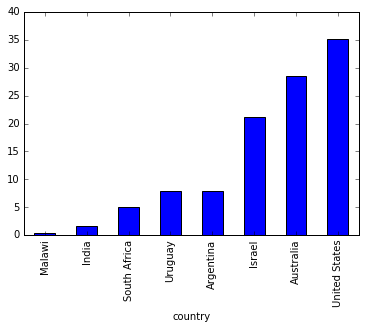## Exercises¶

Here are two exercises. Feel free to consult documentation such as can be found here. The solutions are below. The cell with "solution below" is mean to push them below your line of sight and save you from temptation.

#### Exercise 1¶

Generate 10000 data points from the exponential distribution with density

$$f(x; \alpha) = \alpha \exp(-\alpha x) \qquad (x > 0, \alpha > 0)$$

using scipy.stats and taking $\alpha = 0.5$. Then, after looking up the maximum likelihood estimator of $\alpha$, compute the estimate given your data and check that it is in fact close to $\alpha$.

In :
# Put your solution here


#### Exercise 2¶

Using the same data set, implement maximum likelihood again, but this time pretending that you don't know the analytical expression for the maximum likelihood estimator. Set up the log likelihood function and maximize it numerically using a routine from scipy.optimize.

In :
# Put your solution here


## Solutions¶

In :
# Print some nonsense to partially hide solutions
filler_text = "solution below\n" * 25
print(filler_text)

solution below
solution below
solution below
solution below
solution below
solution below
solution below
solution below
solution below
solution below
solution below
solution below
solution below
solution below
solution below
solution below
solution below
solution below
solution below
solution below
solution below
solution below
solution below
solution below
solution below



#### Solution to Exercise 1¶

After checking the docs for the exponential distribution we proceed as follows

In :
from scipy.stats import expon
alpha = 0.5
n = 10000
ep = expon(scale=1.0/alpha)  # scale controls the exponential parameter
x = ep.rvs(n)


Let's check we've got the right distribution here

In :
fig, ax = plt.subplots(figsize=(8, 5))
xmin, xmax = 0.001, 10.0
ax.set_xlim(xmin, xmax)
ax.hist(x, normed=True, bins=40, alpha=0.3)
grid = np.linspace(xmin, xmax, 200)
ax.plot(grid, ep.pdf(grid), 'g-', lw=2, label='true density')
ax.legend()

Out:
<matplotlib.legend.Legend at 0x7f77a2118048>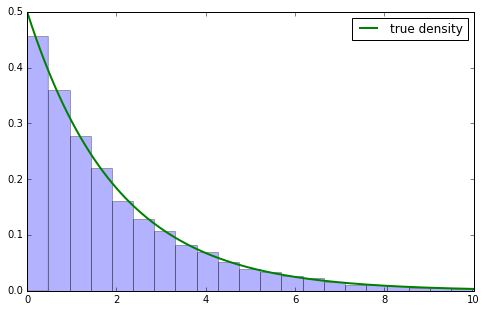It's well-known that the MLE of $\alpha$ is $1/\bar x$ where $\bar x$ is the mean of the sample. Let's check that it is indeed close to $\alpha$.

In :
alpha_mle = 1.0 / x.mean()
print("max likelihood estimate of alpha is {}".format(alpha_mle))

max likelihood estimate of alpha is 0.5042739418869412

In :
s = x.sum()
def neg_loglike(a):
"Minus the log likelihood function for exponential"
return - n * np.log(a) + a * s


Minimize over a reasonable parameter space

In :
from scipy.optimize import fminbound
fminbound(neg_loglike, 0.01, 10.0)

Out:
0.5042730725954131

This is very close to the analytical value of the max likelihood estimator we got in exercise 1

In [ ]: